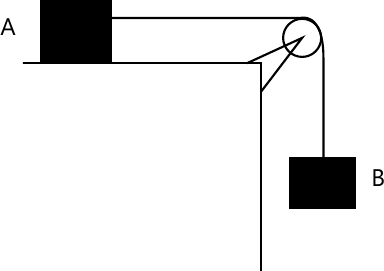# Engineering Mechanics Question paper December 2013

Engineering Mechanics Question paper December 2013 – The first year F.E Engineering mechanics question paper of December 2013 for mumbai university students is common for all students doing semester 1.

### Engineering Mechanics Question paper December 2013

1 (a) A ring is pulled by three forces as shown in figure. Find the forces F and the angle ? if resultant of these three forces is 100 N acting in vertical direction.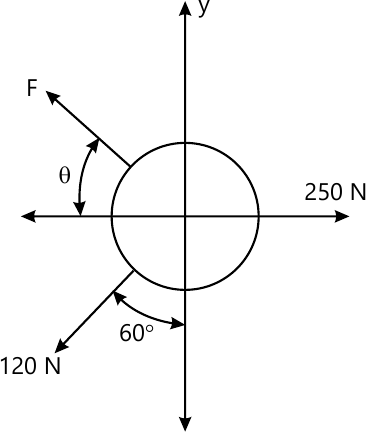4 M
1 (b) State and prove Lami’s Theorem.
4 M
1 (c) Laws of friction.
4 M
1 (d) A motorist is travelling at 90 kmph, when he observes a traffic signal 250 m ahead of him turns red. The traffic signal is timed to stay red for 12 sec. If the motorist wishes to pass the signal without stopping just as it turns green. Determine
(i) The required uniform deceleration of the motor.
(ii) The speed of motor as it passes the signal .
4 M
1 (e) A 50 kg block kept on a 15? inclined plane is pushed down the plane with an initial velocity of 20 m/s. If ?k = 0.4, determine the distance traveled by the block and the time it will take as it comes to rest.
4 M
2 (a) A member ABC is loded by distributed load and pure moment as shown in the Figure. Find the (i) magnitude and (ii) position along AC of the resultant.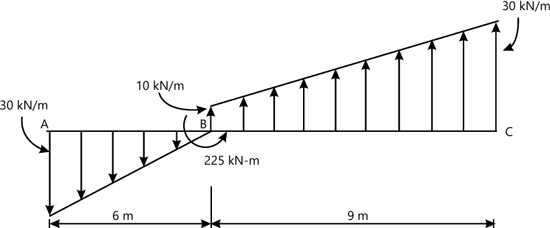6 M
2 (b) A cylinder weighing 1000 N and 1.5 m diameter is supported by a beam AB of length 6 m and weight 400 N as shown in figure. Neglecting friction at the surfaces of contacts, determine (i) Wall reaction at D. (ii) Tension in the cable BC and (iii) Hinged reaction at support A.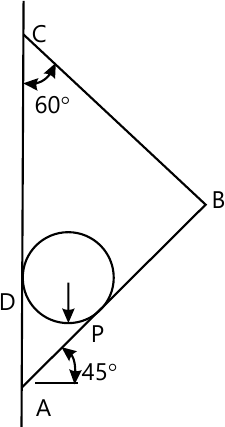8 M
2 (c) A particle of mass 1 kg is acted upon by a force F which varies as shown in figure. If initial velocity of the particle is 10 m/s determine (I) what is the maximum velocity attaind by the particle. (ii) Time when particle will be at the point of reversal.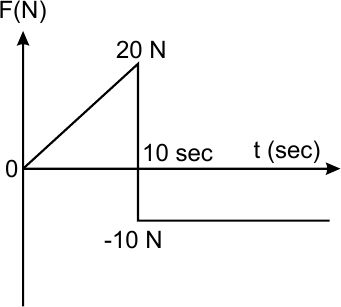6 M
3 (a) Locate the centroid of the shadded area.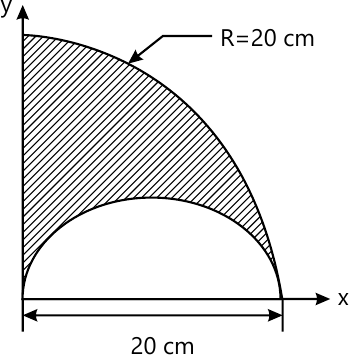8 M
3 (b) A pole is held in place by three cables. If the forces of each cable acting on the pole is as shown in figure determine the resultant.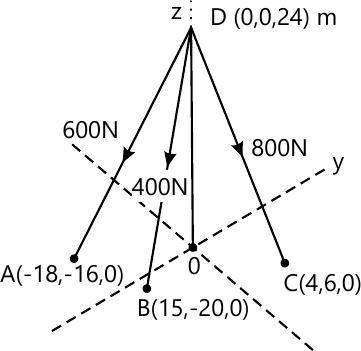6 M
3 (c) Two blocks mA=10 kg and mB=5 kg are connected with cord and pulley system as shown in figure. Determine the velocity of each block when system is started from rest and block B gets displacement by 2 m. Take ?K=0.2 between block A and Horizontal surface.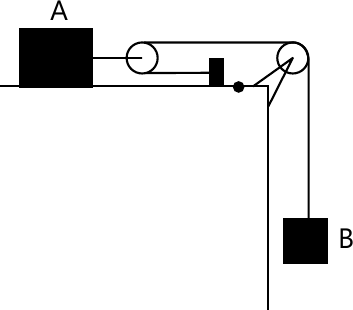6 M
4 (a) Find the support reaction at A and B for the beam showing in figure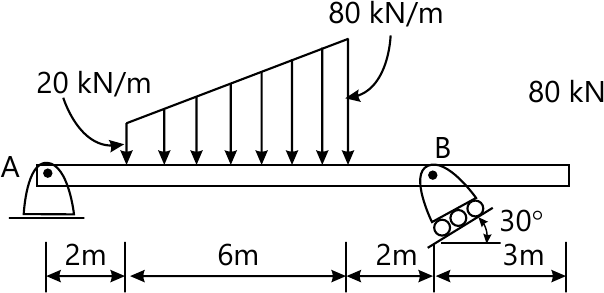8 M
4 (b) A ball is thrown from horizontal level, such that it clears a wall 6 m high, situated at a horizontal distance of 35 m. If the angle of projection is 60? with respect to the horizontal, what should be the minimum velocity of projection?
6 M
4 (c) C’ is a uniform cylinder to which a rod ‘AB’ pinned at ‘A’ and the other end of the rod ‘B’ is moving along a vertical wall as shown in figure. If the end ‘B’ of the rod is moving upward along the wall at a speed of 3.3 m/s find the angular velocity of the cylinder assuming that it is rolling without slipping.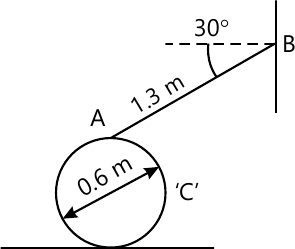6 M
5 (a) Find the forces in members BD, BE and CE by method of section only for the truss shown in the figure. Also find The forces in other members by method of joints.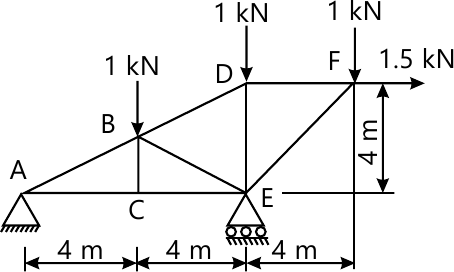8 M
5 (b) In Asian games, for 100 m event an athlete accelerates uniformly from the start to his maximum velocity in a distance of 4 m and runs the remaining distance with that velocity. If the athlete finishes the race in 10.4 sec, determine (i) his initial acceleration, (ii) his maximum velocity.
6 M
5 (c) In figure collar C slides on a horizontal rod. In the position shown rod AB is horizontal and has angular velocity of 0.6 rad/sec clockwise. Determine angular velocity of BC and velocity of collar C.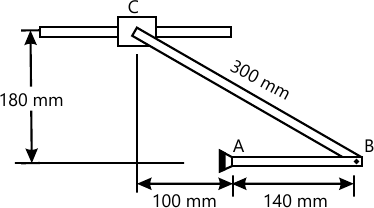6 M
6 (a) A force of 10 kN acts at a point P(2,3,5) m and has its line of action passing through Q(10,-3,4)m. Calculate moment of this force about a point S(1,-10,3)m.
4 M
6 (b) Find the necessary force to raise a heavy stone block of 2000 N. Take coefficient of friction as 0.25 for all surfaces. Neglect the weight of wedge. Take angle of wedge as 15?.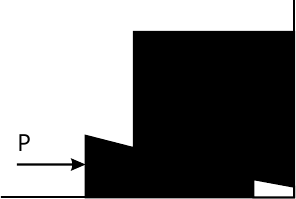8 M
6 (c) A ship A travels in the north making an angle of 45? to the West with a velocity of 18 km/hr and ship B travels in the East with a velocity of 9 km/hr. Find the relative velocity of B w.r.t. ship A.
4 M
6 (d) A body of mass 25 kg resting on a horizontal table is connected by string passing over a smooth pulley at the edge of the table to another body of mass 3.75 kg and hanging vertically as shown. Initially, the friction between the mass A and the table is just sufficient to prevent the motion. If an additional 1.25 kg is added to the 3.75 kg mass, find the acceleration of the masses.